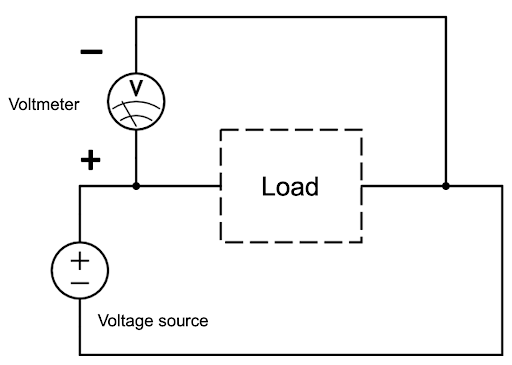# Some uses of a Multimeter

• Measuring current, voltage, resistance etc.
• Test functionality of capacitor.
• Properly checking if a capacitor is full.

# Multimeter Lead Wires

• To ensure the right values are being read attach the lead wires to the proper port of the multimeter.
• Only the red wire switches ports depending on what is being measured and the type of multimeter.
• In the Multimeter shown in Figure 1, while determining the voltage red lead wire will be in port 4 and when determining the current the red lead will be in port 1 or 2, depending on the current source. Utilize port 1 or the 10A port in this case.

# Testing the Multimeter

• To test the functionality of the multimeter, test the continuity. The leads don’t have to be in a specific orientation to test the continuity.
• The continuity can be tested by shifting the dial to the continuity setting shown in Figure 2.
• After the dial is in the continuity setting touch the two leads of the meter together. If there is a sound than the multimeter is working, if there isn’t any sound than the multimeter isn’t functional.

# Measuring Voltage

1. Measure the voltage in PARALLEL. Measuring voltage in series will result in the output from multimeter to be 0.
2. Connect the leads of the multimeter in parallel to the load in the circuit. The connection shown in Figure 3.Figure 3: Multimeter in a Parallel circuit with the load
3. If the voltage shows a negative value than the lead wire orientation is reversed within a circuit. The negative voltage doesn’t affect the data, since it should be viewed as an absolute value. The negative value means the polarity is switched.
4. Turn the dial to the specific range of voltage shown in Figure 4, to get the proper value. For Example: to read a voltage under 2000mV select 2000, Change accordingly.

# Measuring Current

1. NEVER MEASURE CURRENT IN PARALLEL. Measure current in SERIES ONLY.
2. MEASURE IN SERIES. If UNSURE call for help.
3. To measure current, connect the multimeter in series with the load, shown in Figure 5.
4. Select the proper current and adjust the dial accordingly, refer to Figure 4.

# Testing Capacitors

1. To test the capacitors, place the dial into the continuity setting of the multimeter.
2. Touch the leads from the multimeter to lead of the capacitor.
3. If the multimeter makes noise, that means that the capacitor is shorted and is not functional.

# Checking if the Capacitor is fully charged

1. To Check if the capacitor is fully charged the current across the capacitors over time should be checked.
2. The Multimeter must be in SERIES with the capacitor to check the current across the multimeter over time.
3. If there are two capacitors, then both capacitor has to be in series with the power source and the multimeter, to test if the capacitors are full. Following is an example of a one capacitor based circuit to test if it’s fully charged.
4. When the value of the current shown on the multimeter approaches zero, the capacitor is fully charged.